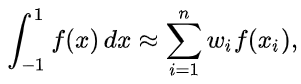In numerical analysis, a quadrature rule is an approximation of the definite integral of a function, usually stated as a weighted sum of a function values at specfied pointswithin the domain of integration. The most common domain of integration for such a rule is taken as [−1,1], so the rule is stated as:which is exact for polynomials of degree 2n-1 or less. This exact rule is known as the Gauss-Legendre quadrature rule.

Initial step - To define the function, let us consider function $y=3x^2-6x$

In :
def function(x):
y= (3*x*x-6*x)
return y


x and w are the gauss points and gauss weights corresponding to the four point gauss quadrature rule

In :
x=[0.339981,-0.339981,0.861136,-0.8661136]
w= [0.652145,0.652145,0.347855,0.347855]


Use "for loop" for computing the integral value of the function

In :
sum_n=0
for i in range(len(x)):
sum_n=sum_n+function(x[i])*w[i]
print(sum_n)

2.0193603541088567